×#### Thank you for registering.

One of our academic counsellors will contact you within 1 working day.

Click to Chat

1800-1023-196

+91-120-4616500

CART 0

• 0

MY CART (5)

Use Coupon: CART20 and get 20% off on all online Study Material

ITEM
DETAILS
MRP
DISCOUNT
FINAL PRICE
Total Price: Rs.

There are no items in this cart.
Continue Shopping• Complete JEE Main/Advanced Course and Test Series
• OFFERED PRICE: Rs. 15,900
• View Details

```Chapter 6: Fractions – Exercise 6.8

Question: 1

Write these fractions appropriately as additions or subtraction: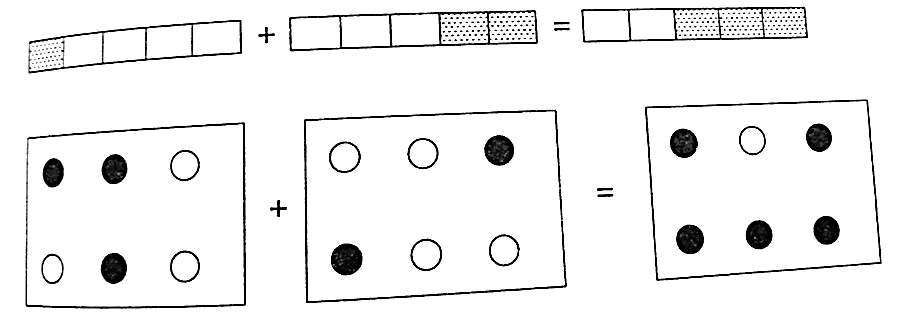Solution:Question: 2

Solve:Solution:

i) The given fractions are:ii) The given fractions are: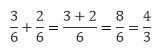iii) the given fractions are:iv) the given fractions are: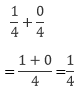v) The given fractions are: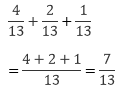vi) the given fractions are: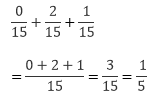vii) the given fractions are: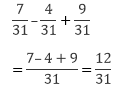viii) the given fractions are: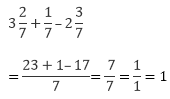Hence the answer is 1/1 = 1

ix) the given fractions are: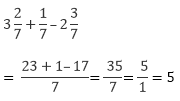Hence the answer is 5/1 = 5

x) the given fractions are: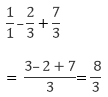xi) the given fractions are: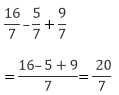Question: 3

Shikha painted 1/5 of the wall space in her room. Her brother ravish helped and painted 3/5 of the wall space. How much did they paint together? How much the room is left unpainted?

Solution:

Shikha painted 1/5 of the wall space in her room

Ravish painted 3/5 of the wall space

Wall space painted by both of them together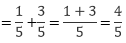Unpainted part of the room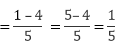Question: 4

Ramesh boughtsugar whereas rohit bought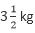of sugar. Find the total amount of sugar bought by both of them.

Solution:

Quantity of sugar bought by ramesh =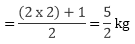Quantity of sugar bought by rohit =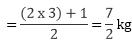Total amount of sugar bought by them:

Quantity of sugar bought by rohit + Quantity of sugar bought by ramesh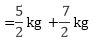= 6 kg (Dividing numerator and denominator by their HCF (6))

Question: 5

The teacher taught 3/5 of the book, Vivek revised 1/5 more on his own. How much does he still have to revise?

Solution:

Fraction of the book taught by the teacher = 3/5

Fraction of the book revised by vivek = 1/5

Fraction of the book still left for revision by vivek:Therefore, Fraction of the book still left for revision by vivek is 2/5

Question: 6

Amit was given 5/7 of a bucket of oranges. What fraction of oranges was left in the basket?

Solution:

Fraction of oranges given to amit = 5/7

Fraction of oranges left in the basket: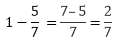Therefore, Fraction of oranges left in the basket is 2/7

Question: 7Solution:

i) Given: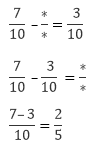Therefore,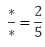ii) Given:Therefore,iii) Given: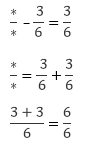Therefore,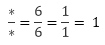iv) Given: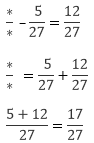Therefore,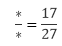```### Course Features

• 728 Video Lectures
• Revision Notes
• Previous Year Papers
• Mind Map
• Study Planner
• NCERT Solutions
• Discussion Forum
• Test paper with Video Solution